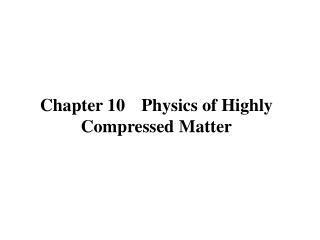Download PresentationChapter 10 Physics of Highly Compressed MatterChapter 10 Physics of Highly Compressed Matter - PowerPoint PPT Presentation

Download PresentationChapter 10 Physics of Highly Compressed Matter
An Image/Link below is provided (as is) to download presentation

Download Policy: Content on the Website is provided to you AS IS for your information and personal use and may not be sold / licensed / shared on other websites without getting consent from its author. While downloading, if for some reason you are not able to download a presentation, the publisher may have deleted the file from their server.

- - - - - - - - - - - - - - - - - - - - - - - - - - - E N D - - - - - - - - - - - - - - - - - - - - - - - - - - -
Presentation Transcript

1. Chapter 10 Physics of Highly Compressed Matter

2. 9.1 Equation of State of Matter in High Pressure

3. More’s QEOS • The pressure is equal to zero at the solid density and the experimental bulk modulus is reproduced. • The cold pressure at the density less than the solid density should be negative (tensile force). • The Fermi pressure of electron is reproduced to be a dominant term at high density in the limit of eF >> Te, wheneFis the Fermi energy of electron. • The ideal gas EOS should be reproduced at high temperature Te >> eF . • The effective charge Z* is determined not only by the thermal ionization, but also by the pressure ionization.

4. Formula of Equation of State Applicable to Wide Range of T and n Total Free Energy Thermodynamic Consistency

5. Ion Equation of State (Cowan Model by More) • 0 < Ti < D (low-temperature solid phase) • D< Ti < Tm (high-temperature solid phase) • Tm < Ti (fluid phase)

6. Electron Equation of State based on Thomas-Fermi Model

7. Thomas Fermi Model Takabe-Takami model,

8. rH is in the unit of g/cm3

9. Bonding Correction where Pb0 = eb0brs/3, rs the solid density, R/Rs = (rs / r)1/3. The parameters eb0 and b are determined so that the total pressure is equal to zero at r = rs and Te = 0 and the bulk modulus defined by

10. Bulk Modulus

11. Equation of State of DD

12. Image of Atoms in Hot-Dense Plasmas(Pressure Ionization) 10.2 Atomic Physics of Hot Dense Plasam

13. Average Atom Model Screened Hydrogen Model rn =a0n2 / Zn

14. photo excitation cross-section sm,m' ∫fm,m'ndn = 1 xn = Pn / gn

15. 10.3 Equation of State Experiments and Planetary Physics

16. Equation of StateGiant Planet